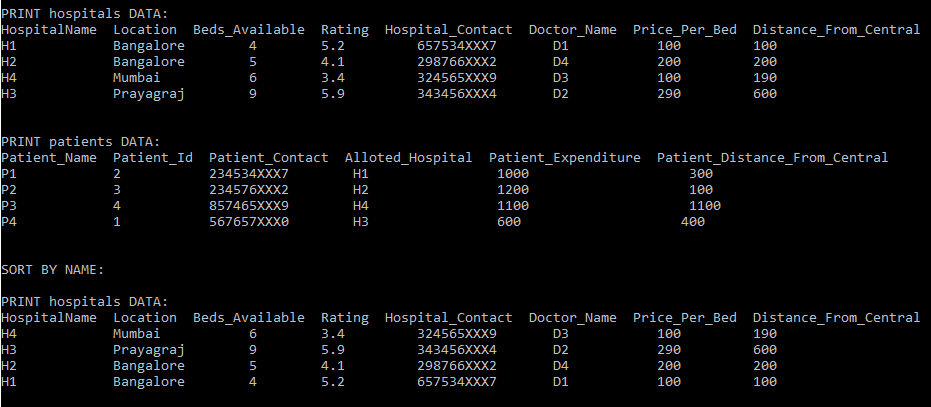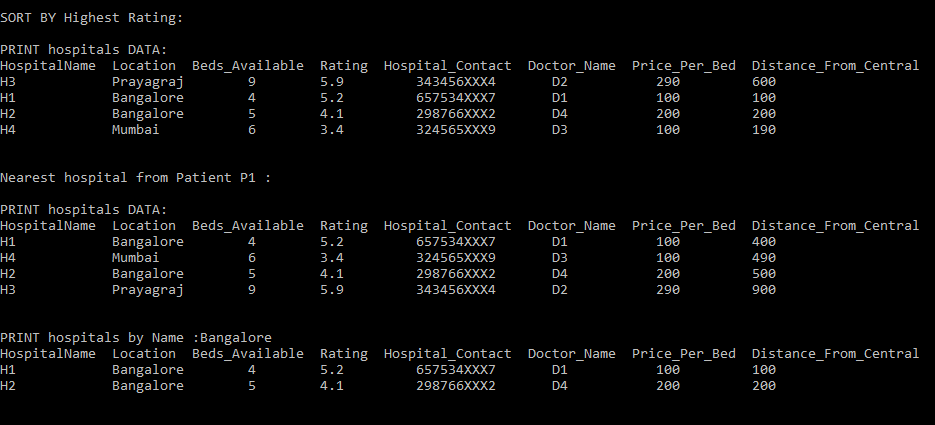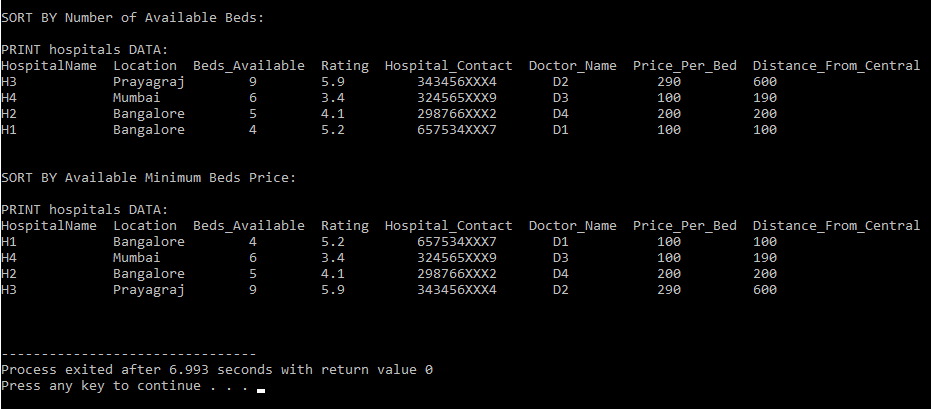# Hospital Management System in C++

• Difficulty Level : Easy
• Last Updated : 18 Jan, 2021

In this article, a C++ program is discussed to manage the Hospital Management System. Given data of Hospitals with the name of hospital, contact and doctors and patients below are the functionalities that needed to be implemented:

Functions Supported:

Want to learn from the best curated videos and practice problems, check out the C++ Foundation Course for Basic to Advanced C++ and C++ STL Course for the language and STL. To complete your preparation from learning a language to DS Algo and many more, please refer Complete Interview Preparation Course.

• Print Hospital DATA
• Print Patients data
• SORT BY Beds Price
• SORT BY Available Beds
• SORT BY NAME
• SORT BY Rating and reviews
• Print hospital of any specific city

The important functions in the program:

1.  PrintHospitalData() : It will print all the hospitals data.
2.  PrintPatientData() : It will print all the hospitals data.
3. SortHospitalByName(): Sort all the hospitals from name
4. SortHospitalByRating(): Sort hospitals according to rating
5. SortByBedsAvailable() : Sort hospitals according to beds available
6. SortByBedsPrice(): Sort hospitals according to the minimum price.

Approach:

• Create classes for both the Hospital dataset and Patient data.
• Initialize variables that store Hospital dataset and Patient data.
• Create Objects for hospitals and Patient classes that access the Hospital dataset and Patient data.
• use two arrays that hold the Hospital dataset and Patient data.
• Implement the given functionality as shown below.

Below is the implementation of the above approach.

## C++

 `// C++ program to implement the Hospital ` `// Management System ` `#include ` `using` `namespace` `std; ` ` `  `// Store the data of Hospital ` `class` `Hospital { ` `public``: ` `    ``string H_name; ` `    ``string location; ` `    ``int` `available_beds; ` `    ``float` `rating; ` `    ``string contact; ` `    ``string doctor_name; ` `    ``int` `price; ` `}; ` ` `  `// Stores the data of Patient ` `class` `Patient : ``public` `Hospital { ` `public``: ` `    ``string P_name; ` `    ``int` `P_id; ` `}; ` ` `  `// Hospital Data ` `void` `PrintHospitalData( ` `    ``vector& hospitals) ` `{ ` `    ``cout << ``"PRINT hospitals DATA:"` `         ``<< endl; ` ` `  `    ``cout << ``"HospitalName     "` `         ``<< ``"Location     "` `         ``<< ``"Beds_Available     "` `         ``<< ``"Rating     "` `         ``<< ``"Hospital_Contact     "` `         ``<< ``"Doctor_Name     "` `         ``<< ``"Price_Per_Bed     \n"``; ` ` `  `    ``for` `(``int` `i = 0; i < 4; i++) { ` `        ``cout << hospitals[i].H_name ` `             ``<< ``"                 "` `             ``<< ``"        "` `             ``<< hospitals[i].location ` `             ``<< ``"           "` `             ``<< hospitals[i].available_beds ` `             ``<< ``"                    "` `             ``<< hospitals[i].rating ` `             ``<< ``"            "` `             ``<< hospitals[i].contact ` `             ``<< ``"             "` `             ``<< hospitals[i].doctor_name ` `             ``<< ``"                  "` `             ``<< ``"        "` `             ``<< hospitals[i].price ` `             ``<< ``"            "` `             ``<< endl; ` `    ``} ` ` `  `    ``cout << endl ` `         ``<< endl; ` `} ` ` `  `// Function to print the patient ` `// data in the hospital ` `void` `PrintPatientData( ` `    ``vector& patients, ` `    ``vector& hospitals) ` `{ ` `    ``cout << ``"PRINT patients DATA:"` `         ``<< endl; ` `    ``cout << ``"Patient_Name     "` `         ``<< ``"Patient_Id     "` `         ``<< ``"Patient_Contact     "` `         ``<< ``"Alloted_Hospital     "` `         ``<< ``"Patient_Expenditure     \n"``; ` ` `  `    ``for` `(``int` `i = 0; i < 4; i++) { ` `        ``cout << patients[i].P_name ` `             ``<< ``"                "` `             ``<< ``"          "` `             ``<< patients[i].P_id ` `             ``<< ``"              "` `             ``<< ``"          "` `             ``<< patients[i].contact ` `             ``<< ``"                   "` `             ``<< hospitals[i].H_name ` `             ``<< ``"                   "` `             ``<< patients[i].price ` `             ``<< ``"            "` `             ``<< endl; ` `    ``} ` ` `  `    ``cout << endl ` `         ``<< endl; ` `} ` ` `  `// Comparator function to sort the ` `// hospital data by name ` `bool` `name(Hospital& A, Hospital& B) ` `{ ` `    ``return` `A.H_name > B.H_name; ` `} ` ` `  `// Function to sort the hospital ` `// data by name ` `void` `SortHospitalByName( ` `    ``vector hospitals) ` `{ ` `    ``// Sort the date ` `    ``sort(hospitals.begin(), ` `         ``hospitals.end(), ` `         ``name); ` ` `  `    ``cout << ``"SORT BY NAME:"` `         ``<< endl ` `         ``<< endl; ` `    ``PrintHospitalData(hospitals); ` `} ` ` `  `// Comparator function to sort the ` `// hospital data by rating ` `bool` `rating(Hospital& A, Hospital& B) ` `{ ` `    ``return` `A.rating > B.rating; ` `} ` ` `  `// Function to sort the hospital ` `// data by namerating ` `void` `SortHospitalByRating(vector hospitals) ` `{ ` `    ``sort(hospitals.begin(), ` `         ``hospitals.end(), ` `         ``rating); ` ` `  `    ``cout << ``"SORT BY Rating:"` `         ``<< endl ` `         ``<< endl; ` ` `  `    ``PrintHospitalData(hospitals); ` `} ` ` `  `// Comparator function to sort the ` `// hospital data by Bed Available ` `bool` `beds(Hospital& A, Hospital& B) ` `{ ` `    ``return` `A.available_beds > B.available_beds; ` `} ` ` `  `// Function to sort the hospital ` `// data by Bed Available ` `void` `SortByBedsAvailable( ` `    ``vector hospitals) ` `{ ` `    ``sort(hospitals.begin(), ` `         ``hospitals.end(), ` `         ``beds); ` ` `  `    ``cout << ``"SORT BY Available Beds:"` `         ``<< endl ` `         ``<< endl; ` ` `  `    ``PrintHospitalData(hospitals); ` `} ` ` `  `// Comparator function to sort the ` `// hospital data by Bed Price ` `bool` `beds_price(Hospital& A, Hospital& B) ` `{ ` `    ``return` `A.price < B.price; ` `} ` ` `  `// Function to sort the hospital ` `// data by Bed Price ` `void` `SortByBedsPrice( ` `    ``vector hospitals) ` `{ ` `    ``sort(hospitals.begin(), ` `         ``hospitals.end(), ` `         ``beds_price); ` ` `  `    ``cout << ``"SORT BY Available Beds Price:"` `         ``<< endl ` `         ``<< endl; ` ` `  `    ``PrintHospitalData(hospitals); ` `} ` ` `  `// Comparator function to sort the ` `// hospital data by City ` `void` `PrintHospitalBycity( ` `    ``string city, vector hospitals) ` `{ ` `    ``cout << ``"PRINT hospitals by Name :"` `         ``<< city << endl; ` ` `  `    ``cout << ``"HospitalName     "` `         ``<< ``"Location     "` `         ``<< ``"Beds_Available     "` `         ``<< ``"Rating     "` `         ``<< ``"Hospital_Contact     "` `         ``<< ``"Doctor_Name     "` `         ``<< ``"Price_Per_Bed     \n"``; ` ` `  `    ``for` `(``int` `i = 0; i < 4; i++) { ` ` `  `        ``if` `(hospitals[i].location != city) ` `            ``continue``; ` `        ``cout << hospitals[i].H_name ` `             ``<< ``"                  "` `             ``<< ``"       "` `             ``<< hospitals[i].location ` `             ``<< ``"           "` `             ``<< hospitals[i].available_beds ` `             ``<< ``"                    "` `             ``<< hospitals[i].rating ` `             ``<< ``"            "` `             ``<< hospitals[i].contact ` `             ``<< ``"             "` `             ``<< hospitals[i].doctor_name ` `             ``<< ``"                "` `             ``<< ``"          "` `             ``<< hospitals[i].price ` `             ``<< ``"            "` `             ``<< endl; ` `    ``} ` `    ``cout << endl ` `         ``<< endl; ` `} ` ` `  `// Function to implement Hospital ` `// Management System ` `void` `HospitalManagement( ` `    ``string patient_Name[], ``int` `patient_Id[], ` `    ``string patient_Contact[], ``int` `bookingCost[], ` `    ``string hospital_Name[], string locations[], ``int` `beds[], ` `    ``float` `ratings[], string hospital_Contact[], ` `    ``string doctor_Name[], ``int` `prices[]) ` `{ ` `    ``// Stores the Hospital data ` `    ``// and user data ` `    ``vector hospitals; ` ` `  `    ``// Create Objects for hospital ` `    ``// and the users ` `    ``Hospital h; ` ` `  `    ``// Initialize the data ` `    ``for` `(``int` `i = 0; i < 4; i++) { ` `        ``h.H_name = hospital_Name[i]; ` `        ``h.location = locations[i]; ` `        ``h.available_beds = beds[i]; ` `        ``h.rating = ratings[i]; ` `        ``h.contact = hospital_Contact[i]; ` `        ``h.doctor_name = doctor_Name[i]; ` `        ``h.price = prices[i]; ` `        ``hospitals.push_back(h); ` `    ``} ` ` `  `    ``// Stores the patient data ` `    ``vector patients; ` `    ``Patient p; ` ` `  `    ``// Initialize the data ` `    ``for` `(``int` `i = 0; i < 4; i++) { ` `        ``p.P_name = patient_Name[i]; ` `        ``p.P_id = patient_Id[i]; ` `        ``p.contact = patient_Contact[i]; ` `        ``p.price = bookingCost[i]; ` `        ``patients.push_back(p); ` `    ``} ` ` `  `    ``cout << endl; ` ` `  `    ``// Call the various operations ` `    ``PrintHospitalData(hospitals); ` `    ``PrintPatientData(patients, hospitals); ` ` `  `    ``SortHospitalByName(hospitals); ` `    ``SortHospitalByRating(hospitals); ` `    ``PrintHospitalBycity(``"Bangalore"``, hospitals); ` `    ``SortByBedsAvailable(hospitals); ` `    ``SortByBedsPrice(hospitals); ` `} ` ` `  `// Driver Code ` `int` `main() ` `{ ` `    ``// Stores hospital data and ` `    ``// the user data ` `    ``string patient_Name[] = { ``"P1"``, ``"P2"``, ``"P3"``, ``"P4"` `}; ` `    ``int` `patient_Id[] = { 2, 3, 4, 1 }; ` `    ``string patient_Contact[] ` `        ``= { ``"234534XXX7"``, ``"234576XXX2"``, ``"857465XXX9"``, ` `            ``"567657XXX0"` `}; ` `    ``int` `bookingCost[] = { 1000, 1200, 1100, 600 }; ` ` `  `    ``string hospital_Name[] = { ``"H1"``, ``"H2"``, ``"H4"``, ``"H3"` `}; ` `    ``string locations[] = { ``"Bangalore"``, ``"Bangalore"``, ` `                           ``"Mumbai   "``, ``"Prayagraj"` `}; ` `    ``int` `beds[] = { 4, 5, 6, 9 }; ` `    ``float` `ratings[] = { 5.2, 4.1, 3.4, 5.9 }; ` `    ``string hospital_Contact[] ` `        ``= { ``"657534XXX7"``, ``"298766XXX2"``, ``"324565XXX9"``, ` `            ``"343456XXX4"` `}; ` `    ``string doctor_Name[] = { ``"D1"``, ``"D4"``, ``"D3"``, ``"D2"` `}; ` `    ``int` `prices[] = { 100, 200, 100, 290 }; ` ` `  `    ``// Function Call ` `    ``HospitalManagement( ` `        ``patient_Name, patient_Id, patient_Contact, ` `        ``bookingCost, hospital_Name, locations, beds, ` `        ``ratings, hospital_Contact, doctor_Name, prices); ` ` `  `    ``return` `0; ` `}`

Output:My Personal Notes arrow_drop_up
Recommended Articles
Page :